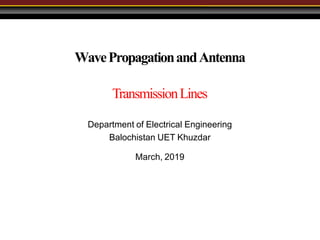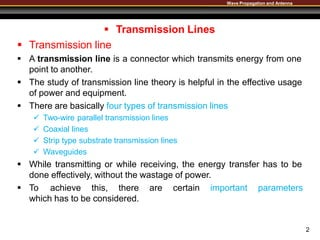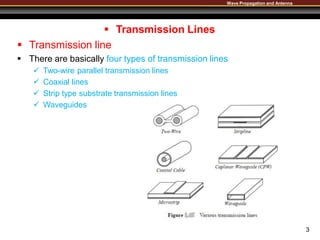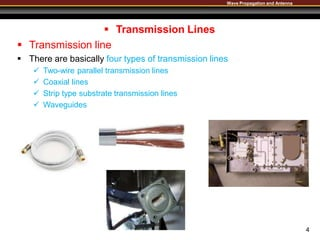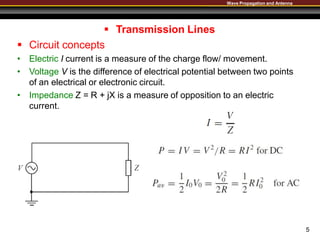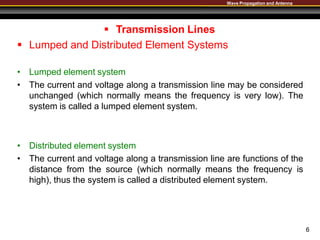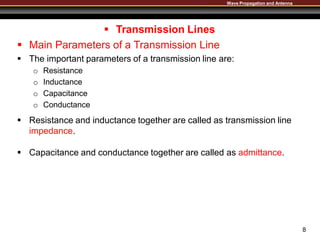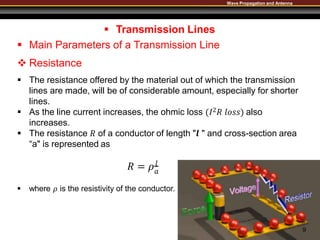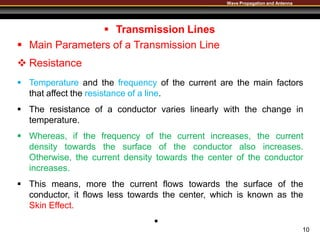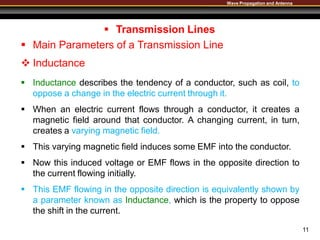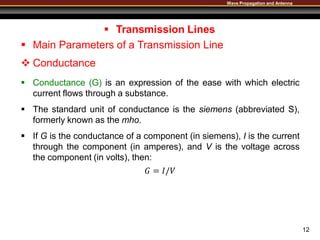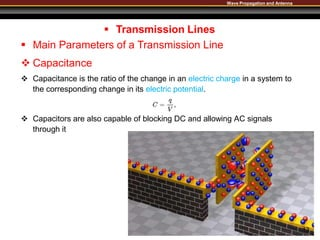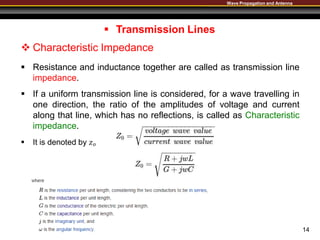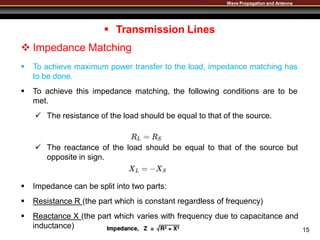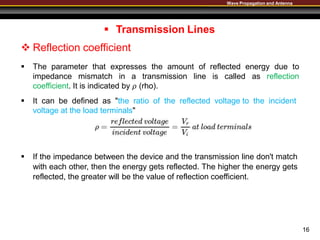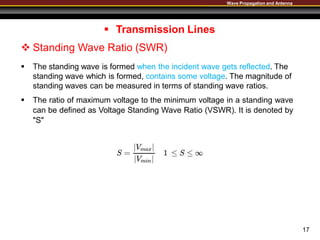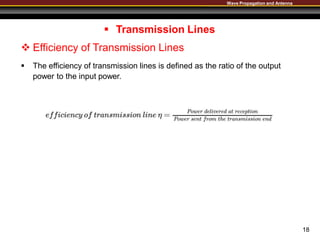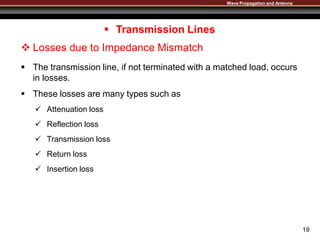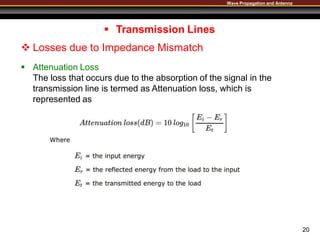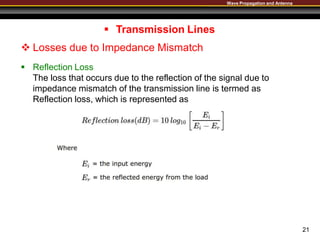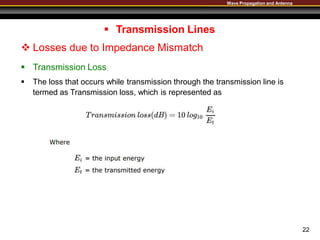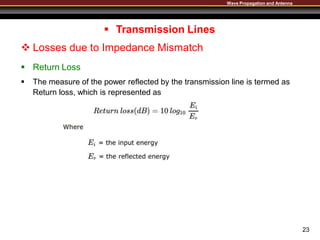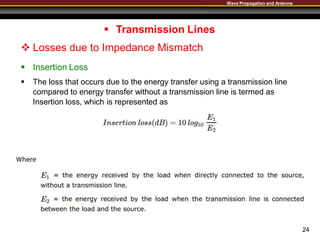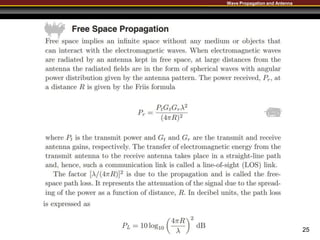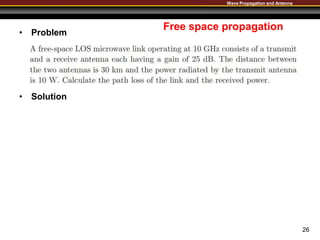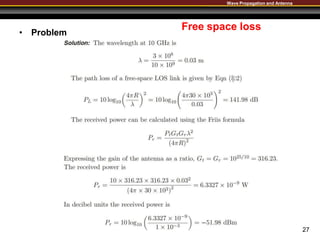1 of 27

### 01 Lecture Transmission Lines 01.pptx

1. WavePropagationandAntenna TransmissionLines Department of Electrical Engineering Balochistan UET Khuzdar March, 2019
2. Wave Propagation and Antenna 2  Transmission Lines  Transmission line  A transmission line is a connector which transmits energy from one point to another.  The study of transmission line theory is helpful in the effective usage of power and equipment.  There are basically four types of transmission lines  Two-wire parallel transmission lines  Coaxial lines  Strip type substrate transmission lines  Waveguides  While transmitting or while receiving, the energy transfer has to be done effectively, without the wastage of power.  To achieve this, there are certain important parameters which has to be considered.
3. Wave Propagation and Antenna  Transmission Lines  Transmission line  There are basically four types of transmission lines  Two-wire parallel transmission lines  Coaxial lines  Strip type substrate transmission lines  Waveguides 3
4. Wave Propagation and Antenna  Transmission Lines  Transmission line  There are basically four types of transmission lines  Two-wire parallel transmission lines  Coaxial lines  Strip type substrate transmission lines  Waveguides 4
5. Wave Propagation and Antenna  Transmission Lines  Circuit concepts • Electric I current is a measure of the charge flow/ movement. • Voltage V is the difference of electrical potential between two points of an electrical or electronic circuit. • Impedance Z = R + jX is a measure of opposition to an electric current. 5
6. Wave Propagation and Antenna 6  Transmission Lines  Lumped and Distributed Element Systems • Lumped element system • The current and voltage along a transmission line may be considered unchanged (which normally means the frequency is very low). The system is called a lumped element system. • Distributed element system • The current and voltage along a transmission line are functions of the distance from the source (which normally means the frequency is high), thus the system is called a distributed element system.
7. Wave Propagation and Antenna  Transmission Lines  Transmission Line Model A distributed element system is converted to a lumped one 7
8. Wave Propagation and Antenna 8  Transmission Lines  Main Parameters of a Transmission Line  The important parameters of a transmission line are: o Resistance o Inductance o Capacitance o Conductance  Resistance and inductance together are called as transmission line impedance.  Capacitance and conductance together are called as admittance.
9. Wave Propagation and Antenna  Transmission Lines  Main Parameters of a Transmission Line  Resistance  The resistance offered by the material out of which the transmission lines are made, will be of considerable amount, especially for shorter lines.  As the line current increases, the ohmic loss (𝐼2𝑅 𝑙𝑜𝑠𝑠) also increases.  The resistance 𝑅 of a conductor of length "𝒍 " and cross-section area “a" is represented as 𝑅 = 𝜌𝑙 𝑎  where 𝜌 is the resistivity of the conductor. 9
10. Wave Propagation and Antenna 10  Transmission Lines  Main Parameters of a Transmission Line  Resistance  Temperature and the frequency of the current are the main factors that affect the resistance of a line.  The resistance of a conductor varies linearly with the change in temperature.  Whereas, if the frequency of the current increases, the current density towards the surface of the conductor also increases. Otherwise, the current density towards the center of the conductor increases.  This means, more the current flows towards the surface of the conductor, it flows less towards the center, which is known as the Skin Effect. 
11. Wave Propagation and Antenna 11  Transmission Lines  Main Parameters of a Transmission Line  Inductance  Inductance describes the tendency of a conductor, such as coil, to oppose a change in the electric current through it.  When an electric current flows through a conductor, it creates a magnetic field around that conductor. A changing current, in turn, creates a varying magnetic field.  This varying magnetic field induces some EMF into the conductor.  Now this induced voltage or EMF flows in the opposite direction to the current flowing initially.  This EMF flowing in the opposite direction is equivalently shown by a parameter known as Inductance, which is the property to oppose the shift in the current.
12. Wave Propagation and Antenna 12  Transmission Lines  Main Parameters of a Transmission Line  Conductance  Conductance (G) is an expression of the ease with which electric current flows through a substance.  The standard unit of conductance is the siemens (abbreviated S), formerly known as the mho.  If G is the conductance of a component (in siemens), I is the current through the component (in amperes), and V is the voltage across the component (in volts), then: 𝐺 = 𝐼/𝑉
13. Wave Propagation and Antenna  Transmission Lines  Main Parameters of a Transmission Line  Capacitance  Capacitance is the ratio of the change in an electric charge in a system to the corresponding change in its electric potential.  Capacitors are also capable of blocking DC and allowing AC signals through it 13
14. Wave Propagation and Antenna  Transmission Lines  Characteristic Impedance  Resistance and inductance together are called as transmission line impedance.  If a uniform transmission line is considered, for a wave travelling in one direction, the ratio of the amplitudes of voltage and current along that line, which has no reflections, is called as Characteristic impedance.  It is denoted by 𝑧𝑜 14
15. Wave Propagation and Antenna  Transmission Lines  Impedance Matching  To achieve maximum power transfer to the load, impedance matching has to be done.  To achieve this impedance matching, the following conditions are to be met.  The resistance of the load should be equal to that of the source.  The reactance of the load should be equal to that of the source but opposite in sign.  Impedance can be split into two parts:  Resistance R (the part which is constant regardless of frequency)  Reactance X (the part which varies with frequency due to capacitance and inductance) 15
16. Wave Propagation and Antenna  Transmission Lines  Reflection coefficient  The parameter that expresses the amount of reflected energy due to impedance mismatch in a transmission line is called as reflection coefficient. It is indicated by 𝜌 (rho).  It can be defined as "the ratio of the reflected voltage to the incident voltage at the load terminals"  If the impedance between the device and the transmission line don't match with each other, then the energy gets reflected. The higher the energy gets reflected, the greater will be the value of reflection coefficient. 16
17. Wave Propagation and Antenna  Transmission Lines  Standing Wave Ratio (SWR)  The standing wave is formed when the incident wave gets reflected. The standing wave which is formed, contains some voltage. The magnitude of standing waves can be measured in terms of standing wave ratios.  The ratio of maximum voltage to the minimum voltage in a standing wave can be defined as Voltage Standing Wave Ratio (VSWR). It is denoted by "S" 17
18. Wave Propagation and Antenna  Transmission Lines  Efficiency of Transmission Lines  The efficiency of transmission lines is defined as the ratio of the output power to the input power. 18
19. Wave Propagation and Antenna 19  Transmission Lines  Losses due to Impedance Mismatch  The transmission line, if not terminated with a matched load, occurs in losses.  These losses are many types such as  Attenuation loss  Reflection loss  Transmission loss  Return loss  Insertion loss
20. Wave Propagation and Antenna  Transmission Lines  Losses due to Impedance Mismatch  Attenuation Loss The loss that occurs due to the absorption of the signal in the transmission line is termed as Attenuation loss, which is represented as 20
21. Wave Propagation and Antenna  Transmission Lines  Losses due to Impedance Mismatch  Reflection Loss The loss that occurs due to the reflection of the signal due to impedance mismatch of the transmission line is termed as Reflection loss, which is represented as 21
22. Wave Propagation and Antenna  Transmission Lines  Losses due to Impedance Mismatch  Transmission Loss  The loss that occurs while transmission through the transmission line is termed as Transmission loss, which is represented as 22
23. Wave Propagation and Antenna  Transmission Lines  Losses due to Impedance Mismatch  Return Loss  The measure of the power reflected by the transmission line is termed as Return loss, which is represented as 23
24. Wave Propagation and Antenna  Transmission Lines  Losses due to Impedance Mismatch  Insertion Loss  The loss that occurs due to the energy transfer using a transmission line compared to energy transfer without a transmission line is termed as Insertion loss, which is represented as 24
25. Wave Propagation and Antenna 25
26. Wave Propagation and Antenna Free space propagation • Problem • Solution 26
27. Wave Propagation and Antenna Free space loss • Problem 27